Wiebe et al. tf3

Wiebe et al. 7nx

Wiebe et al. tdk

Liu and West 8c2

Doucet et al. bmch

figure: Ferrie tb4

Svore et al. 1304.0741

background: neon by Orest Tataryn

background: photo by mattbuck , h/t Wiebe

Isard and Blake cc76f6

# Rejection and Particle Filtering for Hamiltonian Learning

## What is a Hamiltonian?

Lots of meanings and applications in condensed matter, quantum info, etc. In this talk, we consider precisely one:

$$\ket{\psi(t)} = \ee^{\ii H t} \ket{\psi(0)}$$

A Hamiltonian generates dynamics.

Learning Hamiltonians is critical to a range of tasks:

Metrology
Learning magnetic fields, etc.
Calibration
Static field / pulse power / crosstalk, etc.
Debugging/Diagnosis
$T_2$ estimation, other noise finding
Verification/Validation
Analog and digital quantum simulation
### **Example**: Ramsey Estimation ### Suppose $H = \omega \sigma_z / 2$ for some unknown $\omega$. Traditional approach: - Prepare $\ket{+} \propto \ket{0} + \ket{1}$, measure “click” w/ pr.: $\|\bra{+} \ee^{\ii \omega t \sigma_z / 2} \ket{+}\|^2 = \cos^2(\omega t / 2)$. - Repeat for many “shots” to estimate click pr. - Repeat for many times to estimate signal.
You'll get something that looks a bit like this: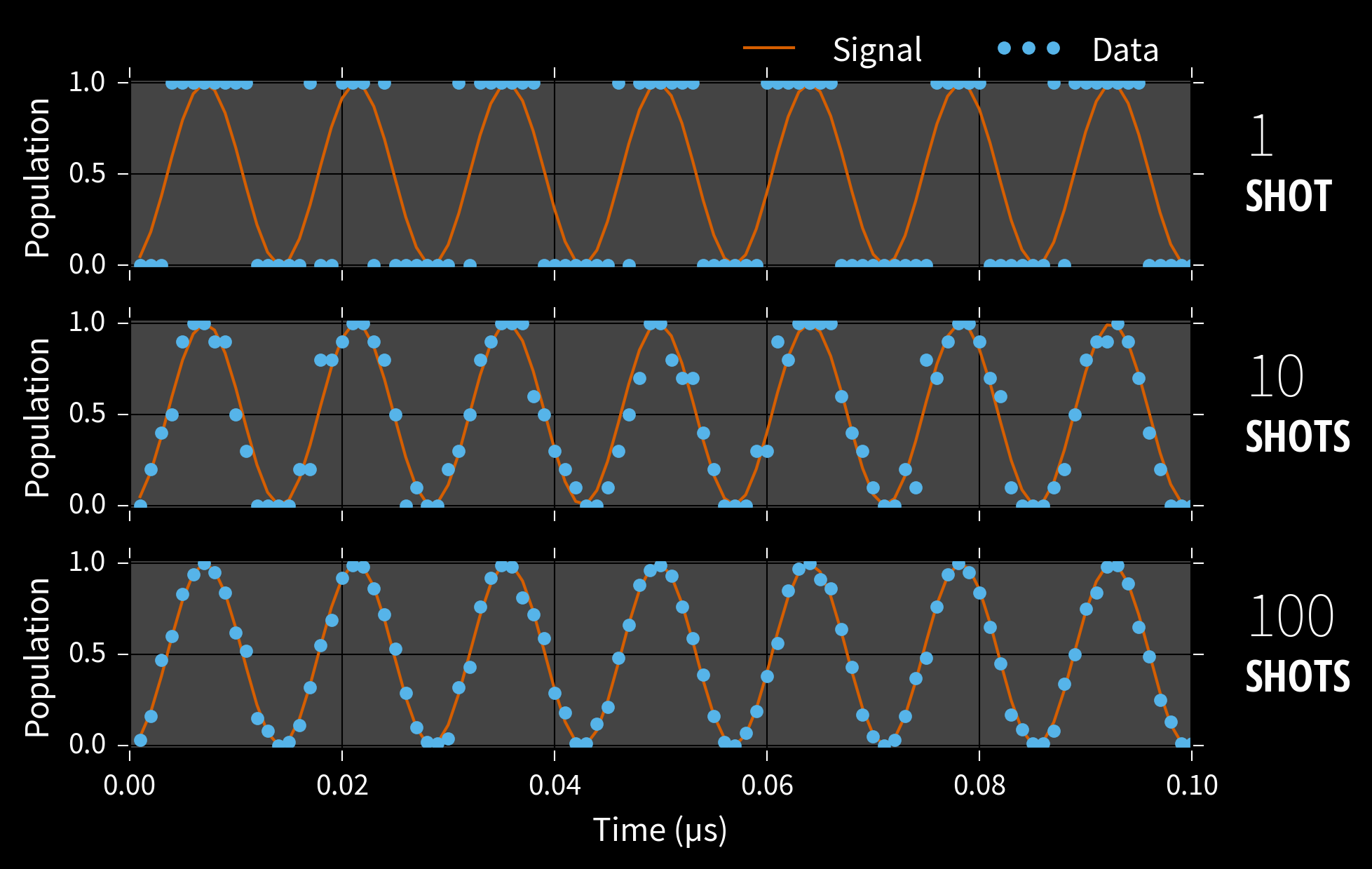What's $\omega$? Fourier transform and look at the peak.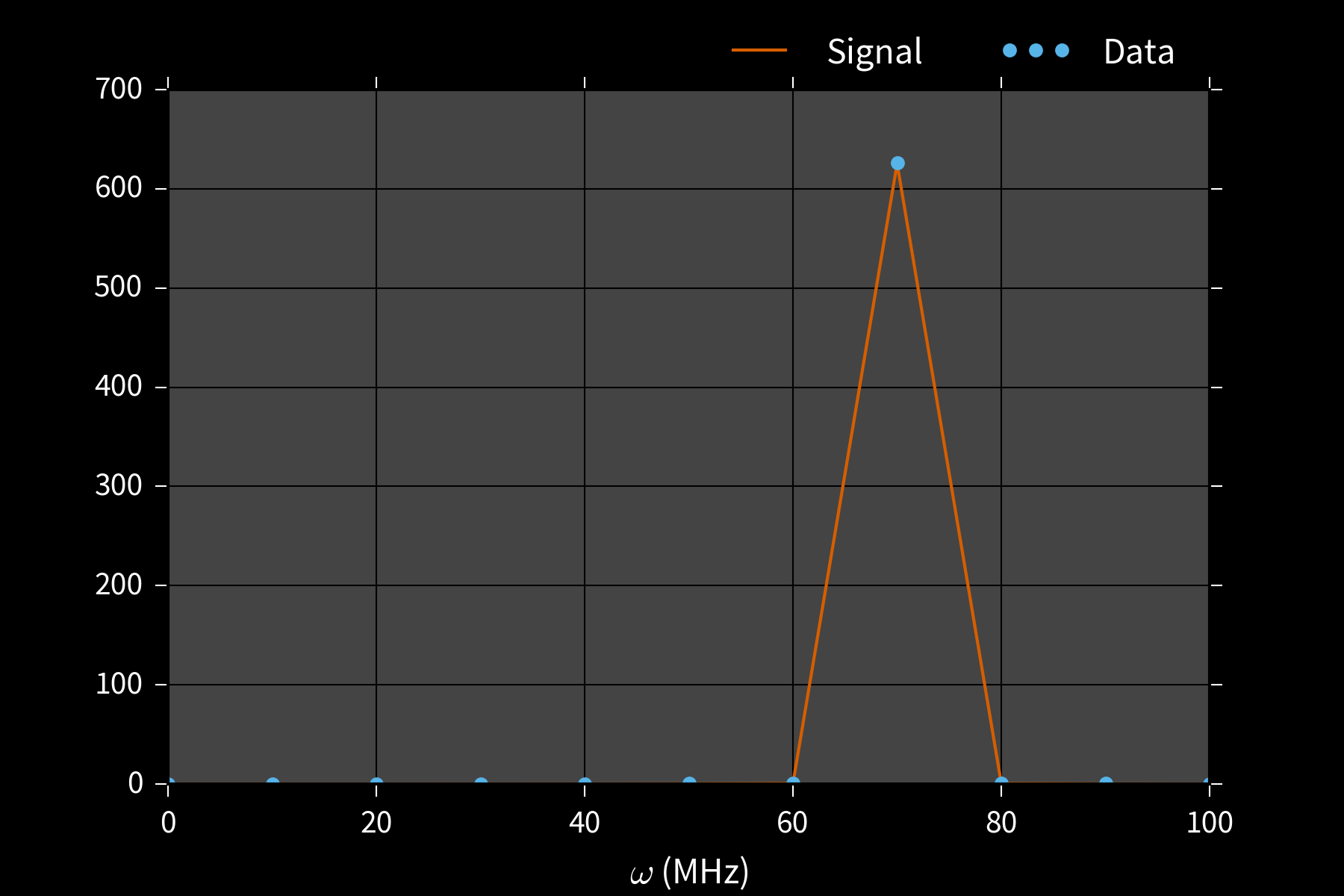We can do better.
# $H = H(\vec{x})$. # Hamiltonian learning is a special case of *parameter estimation*: given data $D$, what is $\vec{x}$?

We want an approach that can work for small and large quantum devices alike.

Punchline: our algorithm can characterize 50-qubit devices.

\begin{align} H & = \sum_{i = 1}^{50} \sum_{j = 1}^{50} \omega_{ij} \sigma_z^{(i)} \sigma_z^{(j)}, \\ \omega_{i,j} & \sim \operatorname{Uniform}\left(0, 10^{-2 (|i - j| - 1)}\right). \end{align}

To get there, we consider several different approaches to parameter estimation.

Analytic Sergeevich et al. c4vv95
Rejection filter Wiebe and Granade bk9d
Particle filter Doucet et al. bmch
Likelihood-free particle filter Ferrie and Granade tdj
Quantum bootstrapping Wiebe, Granade and Cory 7nx
### The Likelihood Function ### $\|\bra{+} \ee^{\ii \omega t \sigma_z / 2} \ket{+}\|^2$ defines probability $\Pr(d | \omega; t)$ for every outcome $d$, model $\omega$ and experiment $t$. Basis for both maximum-likelihood and Bayesian methods.

### Aside: Why Bayesian?

Frequentist methods work. Bayesian methods also work.
Methodology should follow the question of interest.

###### Example
What should I believe the properties of my system are, given my experience and a set of observations?

### Bayesian Parameter Estimation ### The likelihood tells us what we learn from data: $$\Pr(\vec{x} | d; e) = \frac{\Pr(d | \vec{x}; e)}{\Pr(d | e)} \Pr(\vec{x})$$ --- Estimate $\hat{x} = \expect[\vec{x} | d; e] = \int \vec{x} \Pr(\vec{x} | d; e)\dd \vec{x}$. - **Optimal** for mean—squared error.

### Inference as an Iterative Algorithm

Input: Prior $\Pr(\vec{x})$, data set $D$, likelihood $\Pr(d | \vec{x}; e)$

• $p(\vec{x}) \gets \Pr(\vec{x})$
• For each datum $d \in D$ and experiment $e$:
• Update based on $d$:
$p(\vec{x}) \gets \Pr(d | \vec{x}; e) p(\vec{x}) / \Pr(d)$.
• Return $\hat{x} = \int \vec{x}\,p(\vec{x})\,\dd\vec{x}$.
--- At each step, $p(\vec{x})$ also encodes our uncertainty. $\expect[(x - \hat{x})^2 | d; e] = \mathbb{V}[x \mid d; e].$
We can use our posteriors to make *adaptive* decisions: $$e_* = \operatorname{arg min}_e \expect_d[\mathbb{V}(x | d; e)]$$
### **Example**: $x = (\omega)$ ### Can analytically find posterior for Gaussian priors, use to adaptively choose $t_k$. ![](./figures/how-t2.png)

**Problem**: may be intractable to analytically compute $$\hat{x} \defeq \int \Pr(\vec{x} | d; e) \dd\vec{x} = \int \frac{ \Pr(d | \vec{x}; e) }{ \int \Pr(d | \vec{x}; e) \Pr(\vec{x}) \dd\vec{x} } \Pr(\vec{x}) \dd\vec{x}.$$

--- **Answer**: numerically approximate $\int f(\vec{x}) \Pr(\vec{x} | d)\dd\vec{x}$. *Efficient* to use Monte Carlo integration if we can sample from the posterior $\Pr(\vec{x} | d; e)$.
## Rejection Sampling ## Given samples from $\Pr(\vec{x})$ and likelihood function $\Pr(d | \vec{x}; e)$, how do we sample from posterior for datum $d$? - Draw $\vec{x} \sim \Pr(\vec{x})$, accept $\vec{x}$ w/ $\Pr(d | \vec{x}; e)$. Accepted samples are distributed according to posterior.

### Rejection Sampling Isn't Enough

Let $D = {d_1, \dots, d_k}$ be a set of data.

$$\Pr(\text{accept} | \vec{x}) = \Pr(D | \vec{x}) = \prod_{d \in D} \Pr(d | \vec{x}) \overset{k \to \infty}{\longrightarrow} 0.$$

#### Example: Biased Coin $x = (p)$

$\Pr(H | p) = p$, $d \in \{H, T\}$.

$p \approx 0.5 \Longrightarrow \Pr(d_1, \dots, d_k | p) \approx 1 / 2^k$.

We will accept exponentially few samples!

### Gaussian Resampling ### For each datum $d$, use rejection sampling to approximate posterior moments: - $\bar{x} \gets \expect[\vec{x} | d]$. - $\Sigma \gets \operatorname{Cov}[\vec{x} | d] = \expect[\vec{x} \vec{x}^\TT | d] - \bar{x} \bar{x}^\TT$.
--- At the next iteration, draw prior samples from Gaussian with these moments: $$\vec{x} \mid d \sim \mathcal{N}(\bar{x}, \Sigma)$$ Keeps $\Pr(\text{accept}) \approx \text{constant}$.

Can compute $\bar{x}$, $\Sigma$ from one sample at a time by accumulating

$$x_{\Sigma} = \sum x \text{ and } x^2_{\Sigma} = \sum x^2.$$

\begin{align} \bar{x} & = x_{\Sigma} / n_{\text{accept}} \\ \Sigma & = x^2_{\Sigma} / n_{\text{accept}} - \bar{x}^2. \end{align}

Welford's algorithm: numerically-stable modification.

## Rejection Filtering (RejF)

Input: Prior mean $\bar{x}$, prior covariance $\Sigma$, number of samples $m$ to accept.

• For each datum $d$ and experiment $e$:
• $n, \bar{x}', M_2 \gets 0$ Initialize Welford.
• While $n < m$:
• Draw $\vec{x} \sim \mathcal{N}(\bar{x}, \Sigma)$. Sample f/ prior.
• Accept $\vec{x}$ w/ $\Pr(d | \vec{x}; e)$.
• If accepted, update $n$, $\bar{x}'$, $M_2$.
• $\bar{x} \gets \bar{x}'$, $\Sigma \gets M_2 / (n - 1)$. Est. moments.

Easy to implement and embed in control hardware.

### Example: Phase Estimation, $x = (\phi)$

Prepare state $\ket{\phi}$ s. t. $U\ket{\phi} = \ee^{\ii \phi}\ket{\phi}$, measure to learn $\phi$.$\Pr(1 | \phi; M, \theta) = \cos^2(M [\phi - \theta])$

#### Applications

Interferometry / metrology Higgins et al. crwd6w
Gate calibration / robust PE Kimmel et al. bmrg
Quantum simulation and chemistry Reiher et al. 1605.03590

### Example: Phase Estimation, $x = (\phi)$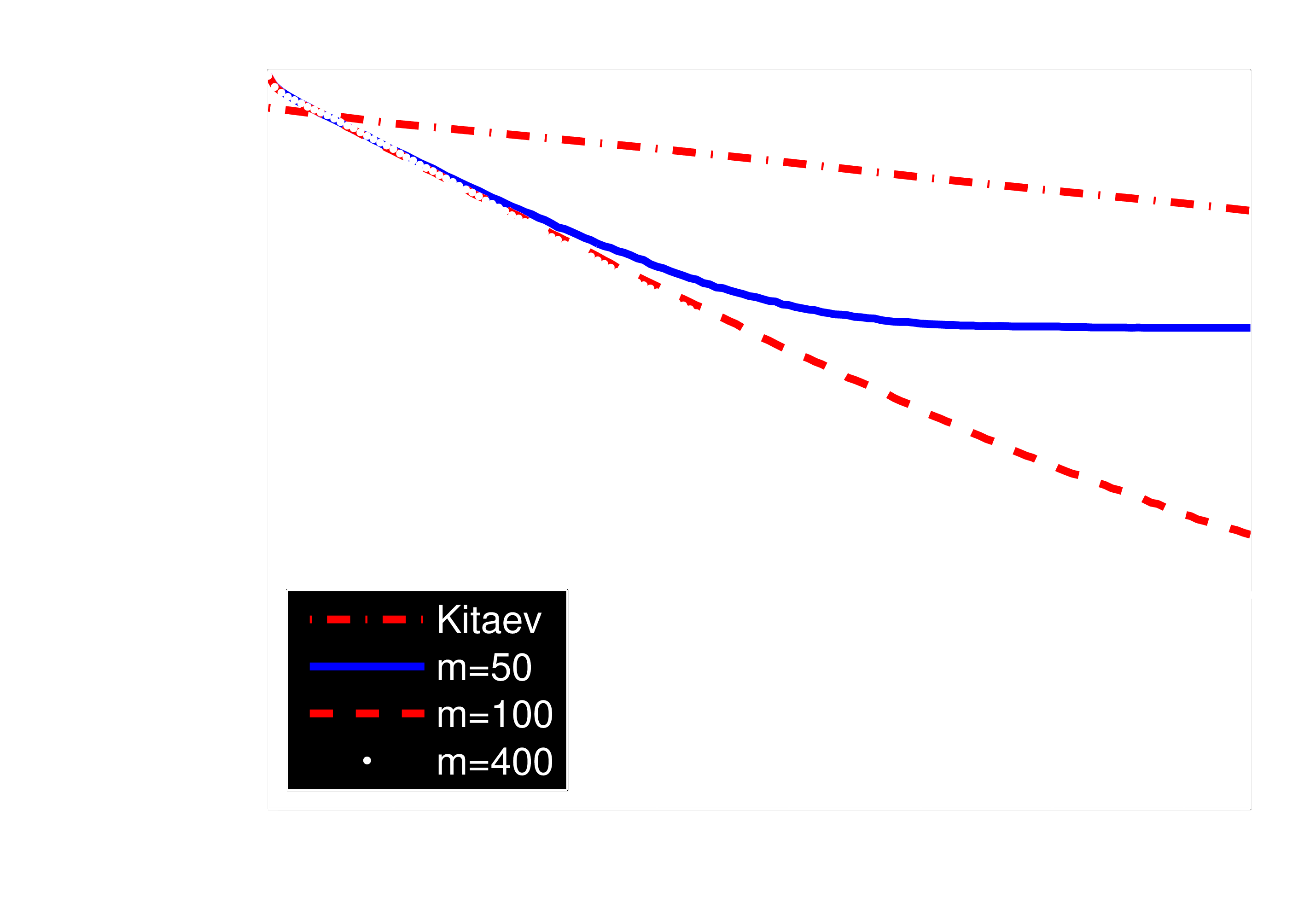**Drawback**: RejF requires posterior after each datum to be $\approx$ Gaussian.

We can solve this by using a more general approach: - Weaken Gaussian assumption. - Generalize the rejection sampling step.

## Liu-West Resampler

If we remember each sample $\vec{x}$, we can use them to relax RejF assumptions.

Input: $a, h \in [0, 1]$ s.t. $a^2 + h^2 = 1$, distribution $p(\vec{x})$.

• Approximate $\bar{x} \gets \expect[\vec{x}]$, $\Sigma \gets \operatorname{Cov}(\vec{x})$
• Draw parent $\vec{x}$ from $p(\vec{x})$.
• Draw $\vec{\epsilon} \sim \mathcal{N}(0, \Sigma)$.
• Return new sample $\vec{x}' \gets a \vec{x} + (1 - a) \bar{x} + h \vec{\epsilon}$.
### Why Does Liu-West Work? ### \begin{align} \vec{x}' & \gets a \vec{x} + (1 - a) \bar{x} + h \vec{\epsilon} \\\\ \expect[\vec{x}'] & = [a + (1 - a)] \bar{x} \\\\ \Cov(\vec{x}') & = (a^2 + h^2) \Cov(\vec{x}). \\\\ \Longrightarrow a^2 + h^2 & = 1 \text{ preserves } \expect[\vec{x}], \Cov(\vec{x}). \end{align} --- Mixes original approximation with $1 - a$ of a Gaussian matching moments. - $a \to 0$: RejF (assumed density) approx - $a \to 1$: Bootstrap - $a = 0.98$: typical case (2% Gaussian).
### From Samples to Particles ### Define a particle $(w_i, \vec{x}_i)$ as a sample $\vec{x}_i$ and a weight $w_i \in [0, 1]$. - $\expect[\vec{x}] = \sum_i w_i \vec{x}_i$ - $\Cov(\vec{x}) = \sum_i w_i \vec{x}_i \vec{x}_i^\TT - \expect[\vec{x}]\expect^\TT[\vec{x}]$ - $\expect[f(\vec{x})] = \sum_i w_i f(\vec{x}_i)$ --- Corresponds to $p(\vec{x}) \approx \sum_i w_i \delta(\vec{x} - \vec{x}_i).$

Particles can represent distributions using either
weights or positions.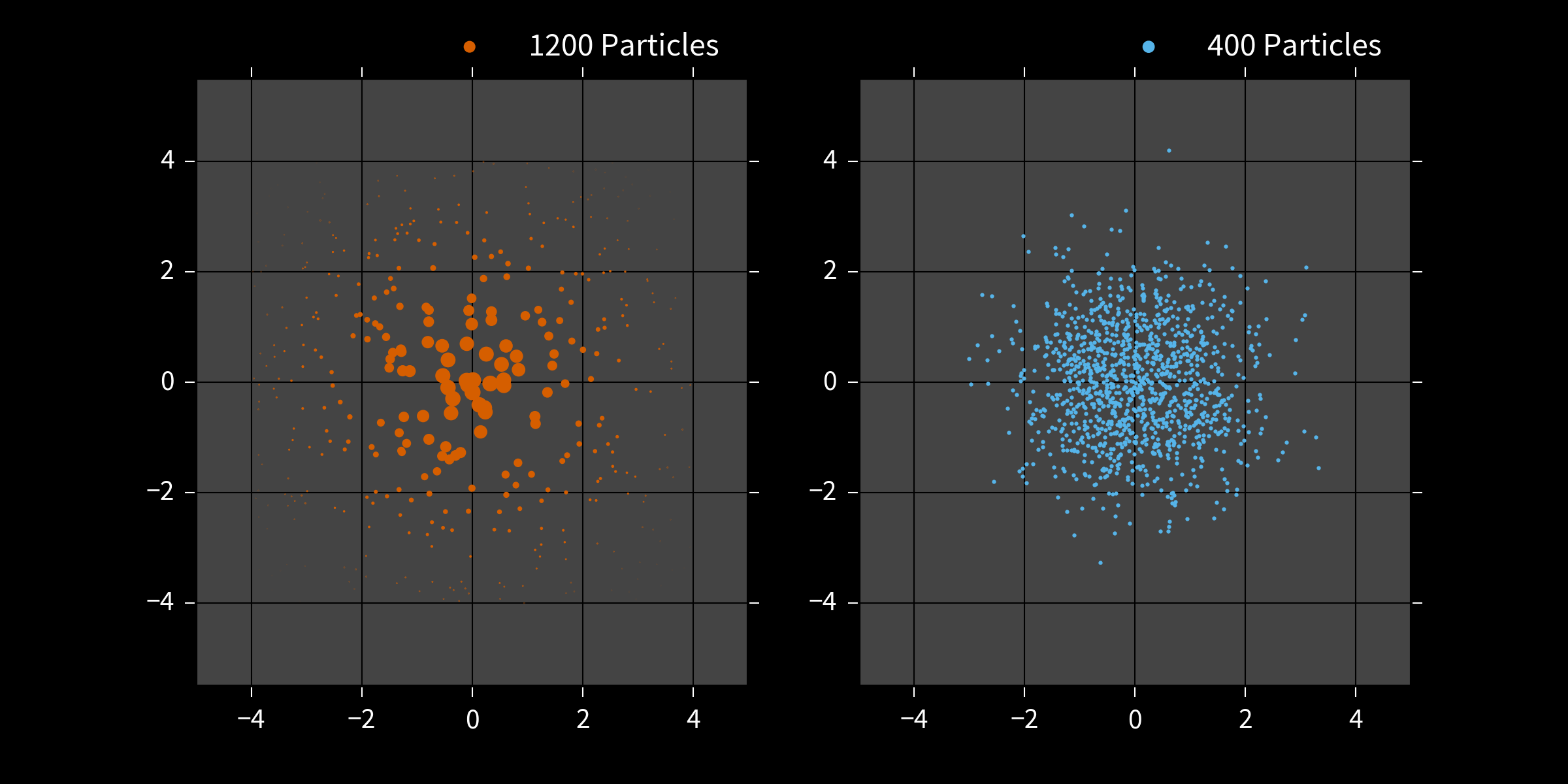## Particle Filter

• Draw $N$ initial samples $\vec{x}_i$ from the prior $\Pr(\vec{x})$ w/ uniform weights.
• Instead of rej. sampling, update weights by \begin{align} \tilde{w}_i & = w_i \times \Pr(d | \vec{x}_i; e) \end{align}
• Renormalize. \begin{align} w_i & \mapsto \tilde{w}_i / \sum_i \tilde{w}_i \end{align}
• Periodically use Liu-West to draw new $\{\vec{x}_i\}$ with uniform weights. Store posterior in positions.

#### Useful for Hamiltonian models...

Rabi/Ramsey/Phase est. Granade et al. s87
Swap spectroscopy Stenberg et al. 7nw

#### ...as well as other QIP tasks.

Tomography Huszár and Holsby s86
Struchalin et al. bmg5
Ferrie 7nt
Randomized benchmarking Granade et al. zmz
Continuous measurement Chase and Geremia chk4q7

### Estimation in Practice

We need a bit more to make particle filtering a practical solution.

• Error bars How well do we know $\vec{x}$?
• Time-dependence $\vec{x} = \vec{x}(t)$
• Software impl. Off-the-shelf.

Dealing with each in turn...

### Error Bars

Particle filters report credible regions $X_\alpha$ s.t. $$\Pr(\vec{x} \in X_\alpha | d; e) \ge \alpha.$$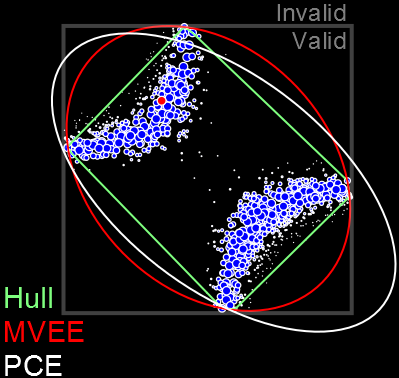E.g.: Posterior covariance ellipse, convex hull, MVEE.

### Time-Dependence

In addition to updating particle weights, move each particle stochastically:

$$\vec{x}(t_{k+1}) = \vec{x}(t_k) + \vec{\eta},\qquad \vec{\eta} \sim \mathcal{N}(0, (t_{k+1} - t_k) \Sigma)$$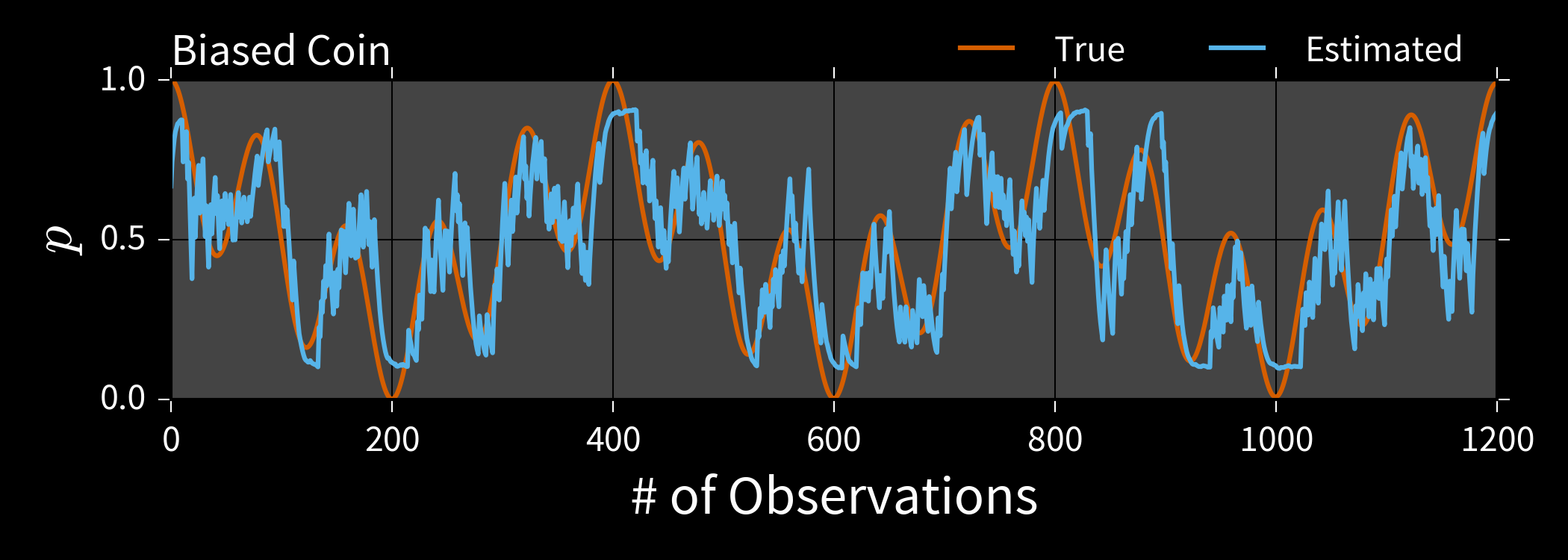### Software Implementation ### We provide **QInfer**, an open-source implementation for use in quantum information. Written in Python, works with MATLAB and Julia.
--- Puts focus on algorithms and experiments, not implementations.

## Bigger and Better

We've seen that filtering is useful for estimating small quantum models. Now let's push on to bigger systems.

What challenges do we face for large systems?

### Simulation Costs

\begin{align} \tilde{w}_i & = w_i \times \color{red}{\Pr(d | \vec{x}_i; e)} \\ w_i & \mapsto \tilde{w}_i / \sum_i \tilde{w}_i \end{align}

- $\Pr(d | \vec{x}_i; e)$ is a *simulation*. - Need to simulate for each particle (~1000s calls/datum). - Infeasible for large quantum models. Let's do better: use *quantum* simulation instead.
### Two Kinds of Simulation ### ![](figures/simulators.png) Weak simulators produce *plausible datasets*.
### Likelihood-Free RejF ### Replace rej. sampling step by drawing datum from likelihood instead of computing exact value: - Draw datum $d'$ from $\Pr(d | \vec{x}; e)$. - Accept $\vec{x}$ if $d = d'$.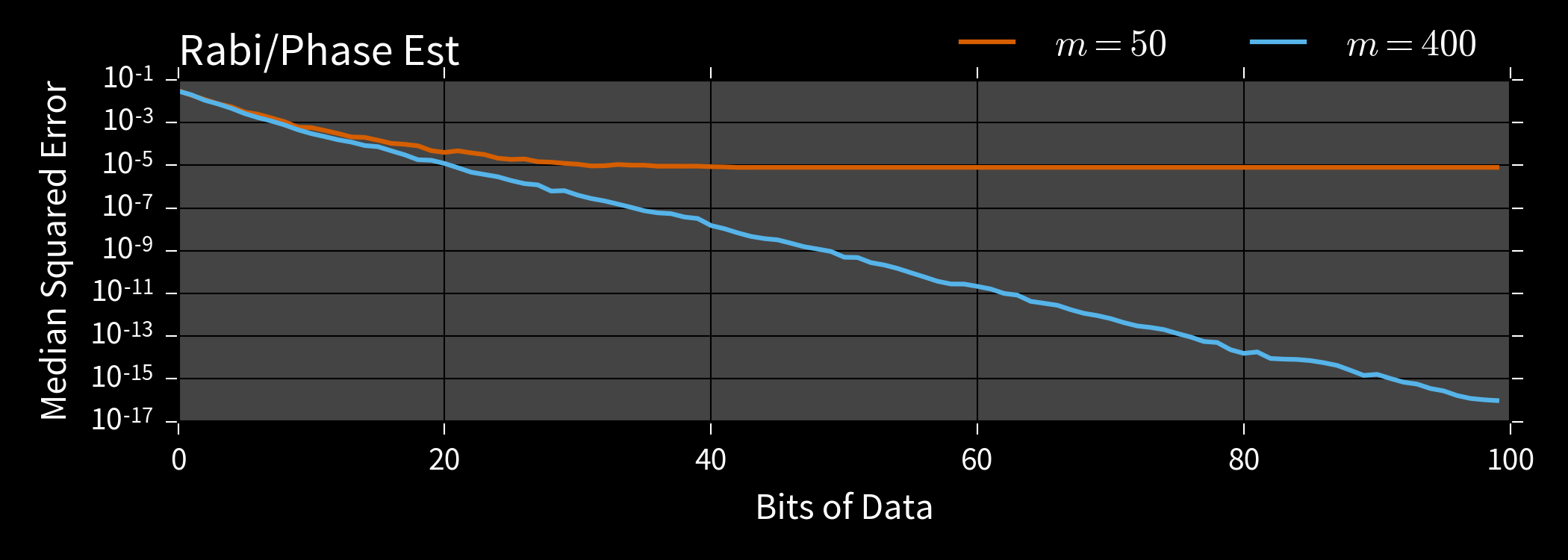### Likelihood-Free Particle Filtering ### Can also use frequencies $f$ from weak simulation to approximate likelihoods in particle filtering. $$\widehat{\Pr}(d | \vec{x}; e) = \frac{f(d | \vec{x}; e)}{k}$$ $k$: number of weak simulation calls used.
### **Example**: Noisy Coin ### How well can we learn the bias $x = (p)$ of a noisy coin? $$\Pr(\text{click} | p) = 0.95 p + 0.1 (1 - p)$$
### Quantum Hamiltonian Learning ### We can learn large Hamiltonians by using likelihood-free filtering w/ interactivity. ![](figures/iqle.png) Perform weak simulations on trusted device only.

### Example: Ising on Complete Graph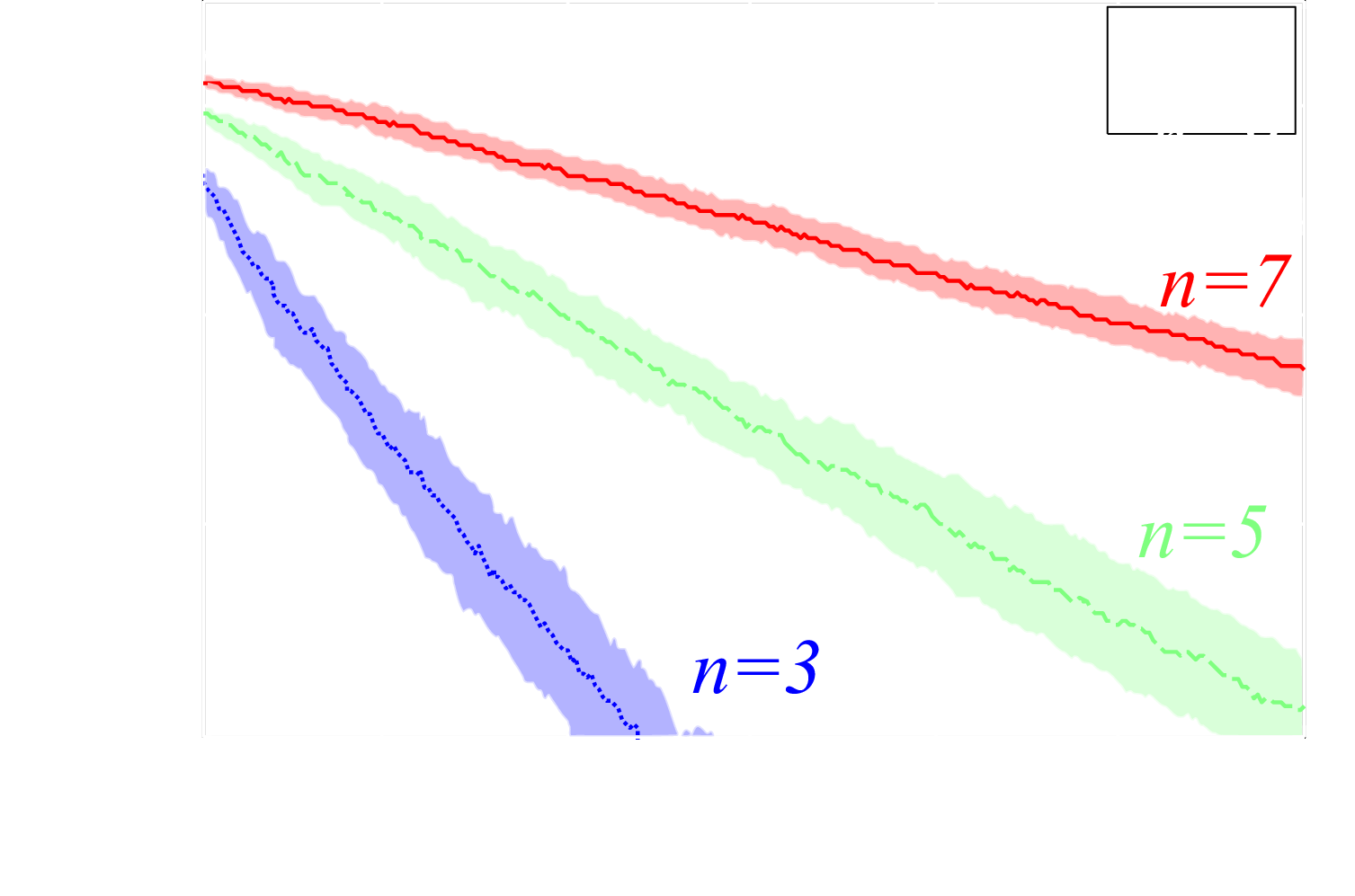Robust even to wrong model. ($0.5$ NN + $10^{-4}$ Complete) ![](figures/qhl-bad-model.png)

### Quantum Bootstrapping

One important approximation f/ physical insight:
information locality.

Allows using small trusted device to learn large Hamiltonians.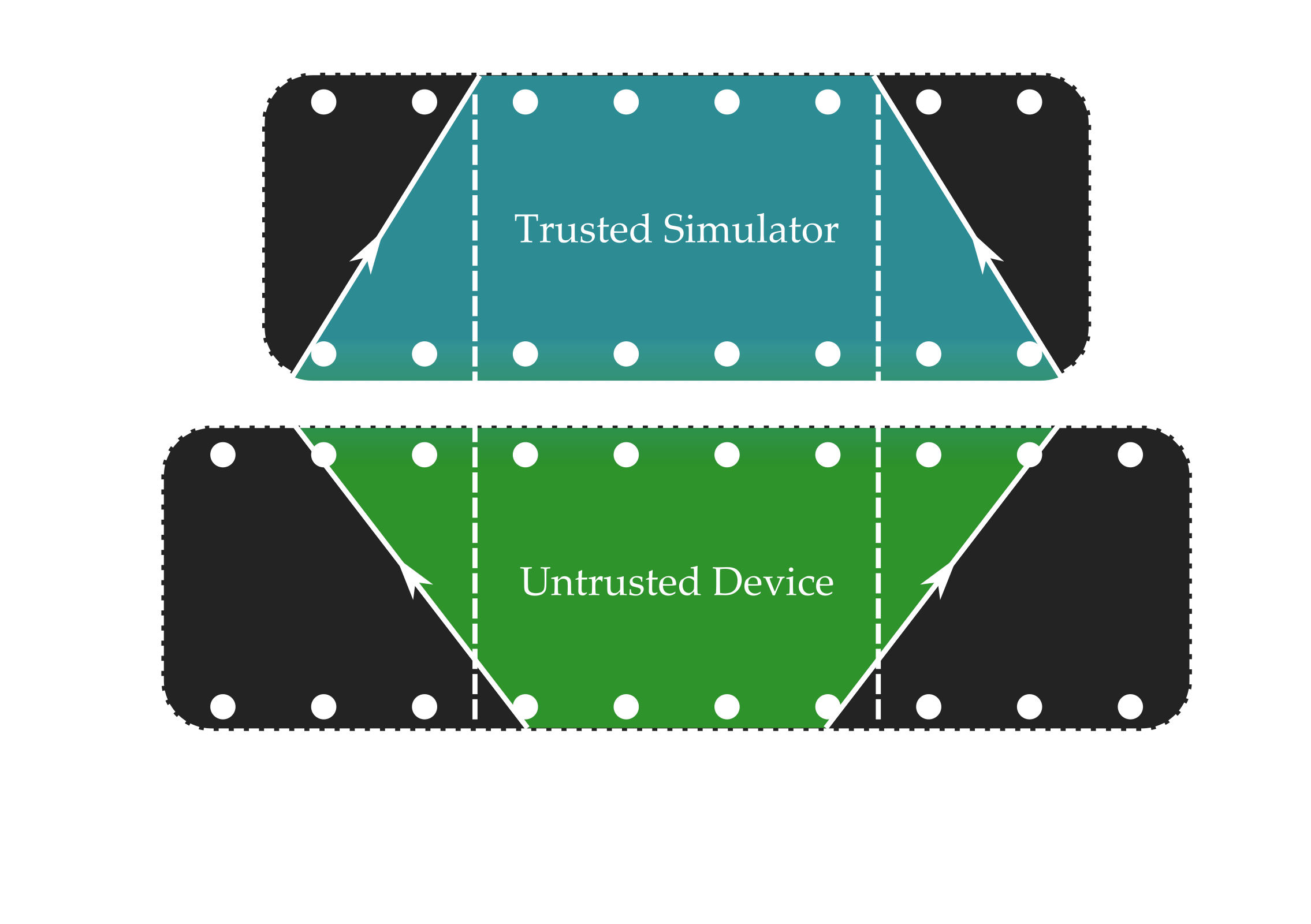Approximation quality can be bounded if Lieb-Robinson velocity is finite.

Scan trusted device across untrusted.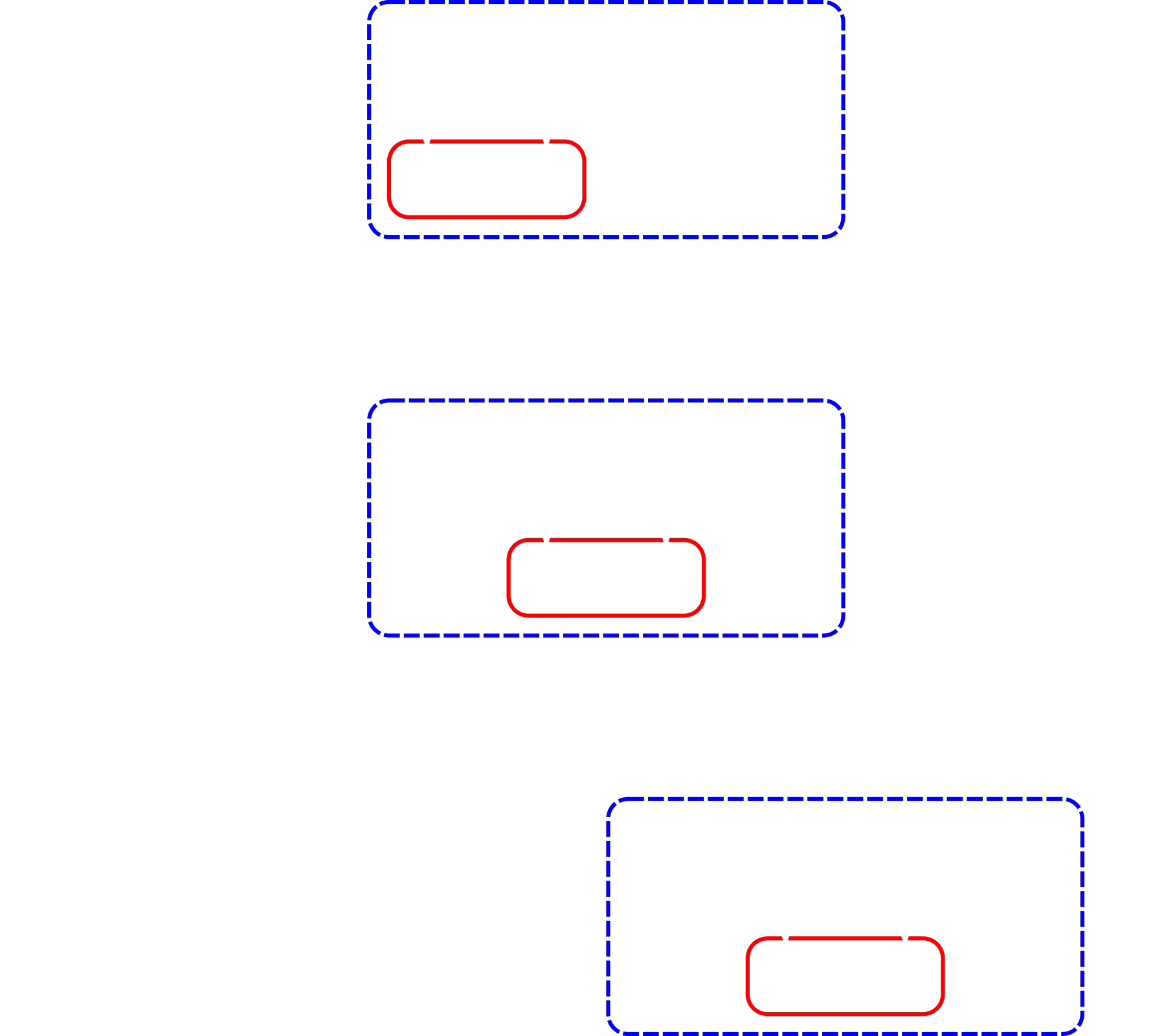Run particle filter only on supported parameters.

#### 50 qubit Ising chain, 8 qubit simulator, 4 qubit observable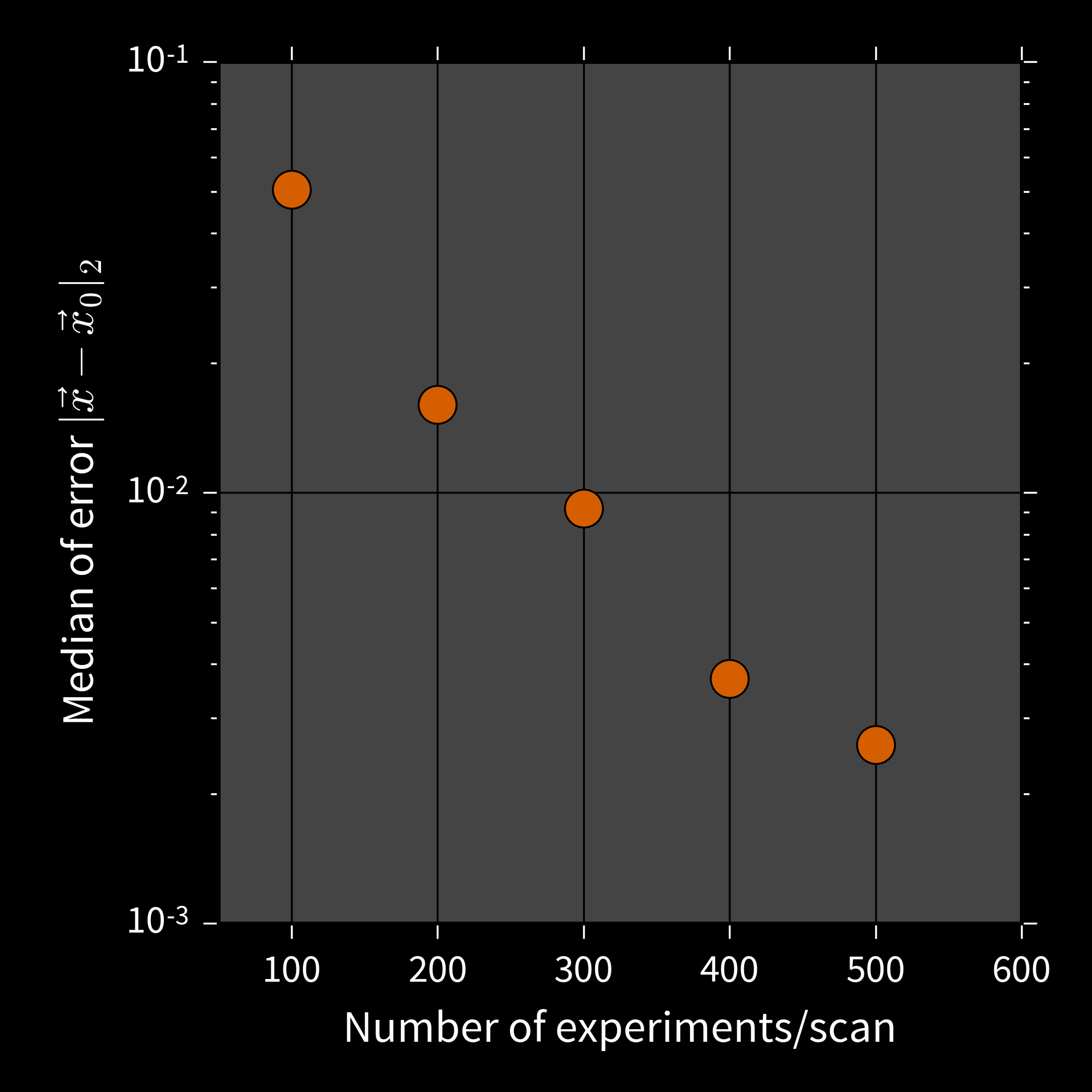### Filtering

• Practical solution for current experimental tasks.
• Enables learning large Hamiltonians using quantum resources.
• Physical insight gives new statistical algorithm for even larger systems.

### Going Further

$\Pr(d | y) = \expect_x[\Pr(d | x) \Pr(x | y)]$.
Allows composing w/ noise, inhomogeneity, etc.
Model selection Ferrie 7nt
Using acceptance ratio or normalizations enables comparing models.
Quantum filtering Wiebe and Granade 1512.03145
Rejection filtering is a dequantization of quantum filtering using Harrow et al. bcz3hc.

# Thank you!

## Welford's Algorithm

Can compute $\bar{x}$, $\Sigma$ from one sample at a time. Numerically stable.

• $n, \bar{x}, M_2 \gets 0$.
• For each sample $x$:
• $n \gets n + 1$ Record # of samples
• $\Delta \gets x - \mu$ Diff to running mean
• $\bar{x} \gets \bar{x} + \Delta / n$ Update running mean
• $M_2 \gets M_2 + \Delta (x - \bar{x})$ Update running var
• Return mean $\bar{x}$, variance $M_2 / (n - 1)$.

Vector case is similar.

We design experiments using the

### PGH: Particle Guess Heuristic

• Draw $\vec{x}_-, \vec{x}_-'$ from current posterior.
• Let $t = 1 / |\vec{x}_- - \vec{x}_-'|$.
• Return $e = (\vec{x}_-, t)$.

$t |\vec{x}_- - \vec{x}_-'| \approx\,$ constant.